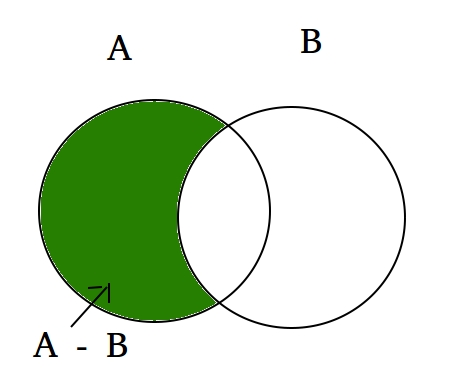Open In App

# Python Set | difference()

The difference between the two sets in Python is equal to the difference between the number of elements in two sets. The function difference() returns a set that is the difference between two sets. Let’s try to find out what will be the difference between two sets A and B. Then (set A – set B) will be the elements present in set A but not in B and (set B – set A) will be the elements present in set B but not in set A.

Example:

```set A = {10, 20, 30, 40, 80}
set B = {100, 30, 80, 40, 60}

set A - set B = {10, 20}
set B - set A = {100, 60}

Explanation: A - B is equal to the elements present in A but not in B
B - A is equal to the elements present in B but not in A```

Let’s look at the Venn diagram of the following difference set function.Syntax:

```set_A.difference(set_B) for (A - B)
set_B.difference(set_A) for (B - A)```

In this program, we will try to find out the difference between two sets set_A and set_B, both the way:

## Python3

 `# Python code to get the difference between two sets``# using difference() between set A and set B` `# Driver Code``A ``=` `{``10``, ``20``, ``30``, ``40``, ``80``}``B ``=` `{``100``, ``30``, ``80``, ``40``, ``60``}``print` `(A.difference(B))``print` `(B.difference(A))`

Output:

```{10, 20}
{100, 60}```

We can also use – operator to find the difference between two sets.

## Python3

 `# Python code to get the difference between two sets``# using difference() between set A and set B` `# Driver Code``A ``=` `{``10``, ``20``, ``30``, ``40``, ``80``}``B ``=` `{``100``, ``30``, ``80``, ``40``, ``60``}``print` `(A ``-` `B)``print` `(B ``-` `A)`

Output:

```{10, 20}
{100, 60}```

If we have equal sets then it will return the null set.

## Python3

 `# Python code to get the difference between two sets``# using difference() between set A and set B` `# Driver Code``A ``=` `{``10``, ``20``, ``30``, ``40``, ``80``}``B ``=` `{``10``, ``20``, ``30``, ``40``, ``80``, ``100``}``print` `(A ``-` `B)`

Output:

`set()`